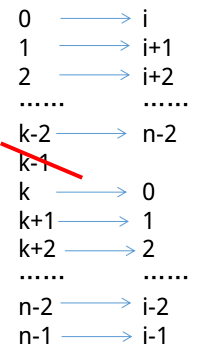# UVA 1394 And Then There Was One / Gym 101415A And Then There Was One / UVAlive 3882 And Then There Was One / POJ 3517 And Then There Was One / Aizu 1275 And Then There Was One （动态规划，思维题）

### Description

Let’s play a stone removing game.

Initially, n stones are arranged on a circle and numbered 1, …, n clockwise (Figure 1). You are also given two numbers k and m. From this state, remove stones one by one following the rules explained below, until only one remains. In step 1, remove stone m. In step 2, locate the k-th next stone clockwise from m and remove it. In subsequent steps, start from the slot of the stone removed in the last step, make k hops clockwise on the remaining stones and remove the one you reach. In other words, skip (k − 1) remaining stones clockwise and remove the next one. Repeat this until only one stone is left and answer its number. For example, the answer for the case n = 8, k = 5, m = 3 is 1, as shown in Figure 1.Initial state: Eight stones are arranged on a circle.
Step 1: Stone 3 is removed since m = 3.
Step 2: You start from the slot that was occupied by stone 3. You skip four stones 4, 5, 6 and 7 (since k = 5), and remove the next one, which is 8.
Step 3: You skip stones 1, 2, 4 and 5, and thus remove 6. Note that you only count stones that are still on the circle and ignore those already removed. Stone 3 is ignored in this case.
Steps 4–7: You continue until only one stone is left. Notice that in later steps when only a few stones remain, the same stone may be skipped multiple times. For example, stones 1 and 4 are skipped twice in step 7.
Final State: Finally, only one stone, 1, is on the circle. This is the final state, so the answer is 1.

### Input

The input consists of multiple datasets each of which is formatted as follows.
n k m
The last dataset is followed by a line containing three zeros. Numbers in a line are separated by a single space. A dataset satisfies the following conditions.
2 ≤ n ≤ 10000, 1 ≤ k ≤ 10000, 1 ≤ m ≤ n
The number of datasets is less than 100.

### Output

For each dataset, output a line containing the stone number left in the final state. No extra characters such as spaces should appear in the output.

### Sample Input

8 5 3
100 9999 98
10000 10000 10000
0 0 0

1
93
2019

## 解决思路$F[i]=(F[i-1]+k)\%i$

$f=(f+k)\%i$

## 代码

#include<iostream>
#include<cstdio>
#include<cstdlib>
#include<cstring>
#include<algorithm>
using namespace std;

const int inf=2147483647;

int main()
{
int n,k,m;
while (scanf("%d%d%d",&n,&k,&m)!=EOF)
{
if ((n==0)&&(m==0)&&(k==0))
break;
int f=0;//初始值
for (int i=2;i<=n;i++)//动态转移
f=(f+k)%i;
f=(f+m-k+1)%n;//把开始是m的情况考虑进去
if (f<=0)//取膜后有可能变成负数或0，此时要将其变成正数
f=f+n;
printf("%d\n",f);
}
return 0;
}
posted @ 2017-08-27 19:49 SYCstudio 阅读(...) 评论(...) 编辑 收藏